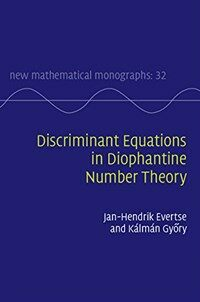> 상세정보

# 상세정보## Discriminant equations in Diophantine number theory (1회 대출)

자료유형
단행본
개인저자
Evertse, J. H. Györy, Kálmán, author.
서명 / 저자사항
Discriminant equations in Diophantine number theory / Jan-Hendrik Evertse, Leiden University, The Netherlands, Kálmán Győry, University of Debrecen, Hungary.
발행사항
Cambridge, United Kingdom :   Cambridge University Press,   c2017.
형태사항
xviii, 457 p. ; 24 cm.
총서사항
New mathematical monographs ;32
ISBN
9781107097612 (hardcover) 1107097614 (hardcover)
서지주기
Includes bibliographical references (p. 440-453) and index.
일반주제명
Diophantine equations. Algebraic number theory. Arithmetical algebraic geometry.
 000 00000cam u2200205 a 4500 001 000045936233 005 20180323110741 008 180322s2017 enk b 001 0 eng d 010 ▼a 2017287147 020 ▼a 9781107097612 (hardcover) 020 ▼a 1107097614 (hardcover) 035 ▼a (KERIS)REF000018300510 040 ▼a YDX ▼b eng ▼c YDX ▼e rda ▼d BNG ▼d OCLCF ▼d SBM ▼d OCL ▼d DLC ▼d 211009 050 0 0 ▼a QA242 ▼b .E938 2017 082 0 4 ▼a 512.74 ▼2 23 084 ▼a 512.74 ▼2 DDCK 090 ▼a 512.74 ▼b E93d 100 1 ▼a Evertse, J. H. 245 1 0 ▼a Discriminant equations in Diophantine number theory / ▼c Jan-Hendrik Evertse, Leiden University, The Netherlands, Kálmán Győry, University of Debrecen, Hungary. 260 ▼a Cambridge, United Kingdom : ▼b Cambridge University Press, ▼c c2017. 300 ▼a xviii, 457 p. ; ▼c 24 cm. 490 1 ▼a New mathematical monographs ; ▼v 32 504 ▼a Includes bibliographical references (p. 440-453) and index. 650 0 ▼a Diophantine equations. 650 0 ▼a Algebraic number theory. 650 0 ▼a Arithmetical algebraic geometry. 700 1 ▼a Györy, Kálmán, ▼e author. 830 0 ▼a New mathematical monographs ; ▼v 32. 945 ▼a KLPA

### 소장정보

No. 소장처 청구기호 등록번호 도서상태 반납예정일 예약 서비스
No. 1 소장처 청구기호 512.74 E93d 등록번호 121243870 도서상태 대출가능 반납예정일 예약 서비스

### 컨텐츠정보

#### 목차

`Preface; Summary; Part I. Preliminaries: 1. Finite etale algebras over fields; 2. Dedekind domains; 3. Algebraic number fields; 4. Tools from the theory of unit equations; Part II. Monic Polynomials and Integral Elements of Given Discriminant, Monogenic Orders: 5. Basic finiteness theorems; 6. Effective results over Z; 7. Algorithmic resolution of discriminant form and index form equations; 8. Effective results over the S-integers of a number field; 9. The number of solutions of discriminant equations; 10. Effective results over finitely generated domains; 11. Further applications; Part III. Binary Forms of Given Discriminant: 12. A brief overview of the basic finiteness theorems; 13. Reduction theory of binary forms; 14. Effective results for binary forms of given discriminant; 15. Semi-effective results for binary forms of given discriminant; 16. Invariant orders of binary forms; 17. On the number of equivalence classes of binary forms of given discriminant; 18. Further applications; Glossary of frequently used notation; References; Index.`

### 관련분야 신착자료

#### Algebra

Aluffi, Paolo (2021)

김대수 (2022)

민만식 (2022)